# What Is A Quintic Function? (3 Key Things To Know)

Quintic functions are used in celestial mechanics to solve for Lagrangian points.  However, there is a lot to know about these fifth degree polynomials, their roots, and their graphs.

So, what is a quintic function?  A quintic function has the form f(x) = ax5 + bx4 + cx3 + dx2 + ex + f, where a, b, c, d, e, & f are real, with a not zero. A quintic function can be one to one or have odd symmetry, but can never have even symmetry. A quintic function has 5 roots, and it can have 0, 2, or 4 complex/imaginary roots.

Of course, a quintic function may have up to four local extrema (maximums or minimums), and it always has an inflection point (since the second derivative is a cubic, or third-degree polynomial, which must always change signs at some point).

In this article, we’ll take a closer look at quintic functions and answer some common questions about them.  We’ll also look at some examples, including graphs of various quintic functions.

Let’s get started.

## What Is A Quintic Function?

A quintic function is one that has the standard form

• f(x) = ax5 + bx4 + cx3 + dx2 + ex + f

where a, b, c, d, e, and f are real, with a not equal to zero.

A quintic function is also called a fifth degree polynomial, or a polynomial function of degree 5.  This means that x5 is the highest power of x that has a nonzero coefficient.

This also means that a quintic polynomial can be described as an odd-degree polynomial.  This means that it will always have at least one real root, and it will always have an odd number of real roots (and an even number of complex roots, which come in conjugate pairs).

The domain of a quintic function is the set of all real numbers.  The range of a quintic function is also the set of all real numbers.

If we set a quintic function equal to zero, we get a quintic equation:

• f(x) = 0

or

• ax5 + bx4 + cx3 + dx2 + ex + f = 0

This equation has 5 solutions.  Some of the solutions may be repeated, and some of them may be complex or imaginary numbers.

## What Does A Quintic Function Look Like?

Here are some examples of quintic functions and their graphs.

### Example 1: Graph Of A Quintic Function With 5 Real Zeros

Consider the quintic function

• f(x) = x5 – 5x3 + 4x

Note that a = 1, b = 0, c = -5, d = 0, e = 4, and f = 0.

We can set this function equal to zero and factor it to find the roots, which will help us to graph it:

• f(x) = 0
• x5 – 5x3 + 4x = 0
• x(x4 – 5x2 + 4) = 0
• x(x2 – 1)(x2 – 4) = 0
• x(x + 1)(x – 1)(x + 2)(x – 2) = 0

So the roots are x = 2, x = 1, x = 0, x = -1, and x = -2.  This means that the graph touches the x-axis in five places: at x = 2, 1, 0, -1, and -2.

We will use these values to help us graph the function: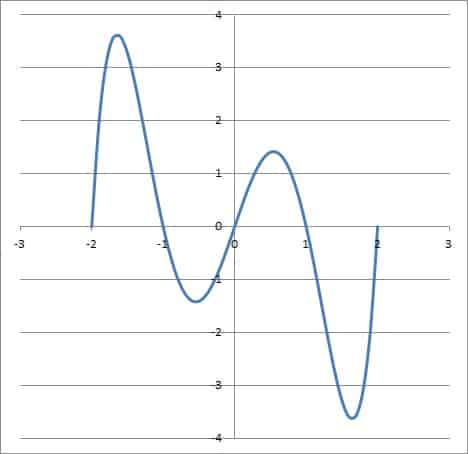The quintic function f(x) = x5 – 5x3 + 4x has zeros at x = 2, 1, 0, -1, and -2.

Note that the function approaches negative infinity as x becomes more negative.  The function approaches positive infinity as x becomes more positive.

### Example 2: Graph Of A Quintic Function With 3 Real Zeros

Consider the quintic function

• f(x) = x5 – x

Note that a = 1, b = 0, c = 0, d = 0, e = -1, and f = 0.

We can set this function equal to zero and factor it to find the roots, which will help us to graph it:

• f(x) = 0
• x5 – x = 0
• x(x4 – 1) = 0
• x(x2 + 1)(x2 – 1) = 0
• x(x + i)(x – i)(x + 1)(x – 1)  [where i = √-1]

So the roots are x = 1, x = 0, x = -1, x = i and x = -i.  So, this quintic only has three real zeros, meaning that the graph only touches the x-axis three times: at x = 1, 0, and -1.

We will use these values to help us graph the function: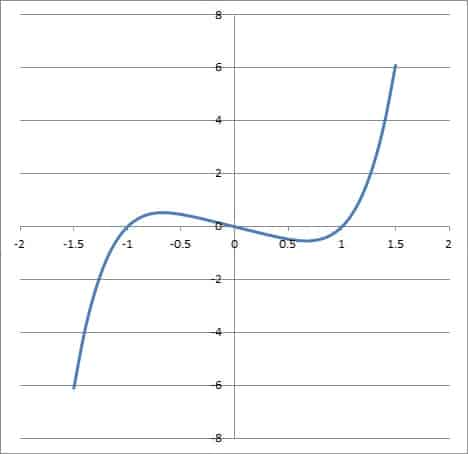The quintic function f(x) = x5 – 5x3 + 4x has zeros at x = 1, 0, -1, i, and-i. The imaginary zeros i and -i do not show up on the graph.

Note that the function approaches negative infinity as x becomes more negative.  The function approaches positive infinity as x becomes more positive.

### Example 3: Graph Of A Quintic Function With 1 Real Zero

Consider the quintic function

• f(x) = x5 + 5x3 + 4x

Note that a = 1, b = 0, c = 5, d = 0, e = 4, and f = 0.

We can set this function equal to zero and factor it to find the roots, which will help us to graph it:

• f(x) = 0
• x5 + 5x3 + 4x = 0
• x(x4 + 5x2 + 4) = 0
• x(x2 + 1)(x2 + 4) = 0
• x(x + i)(x – i)(x + 2i)(x – 2i) = 0

So the roots are x = 2i, x = i, x = 0, x = -i, and x = -2i.  So, this quintic only has one real zero, meaning that the graph only touches the x-axis once: at x = 0.

We will use this to help us graph the function: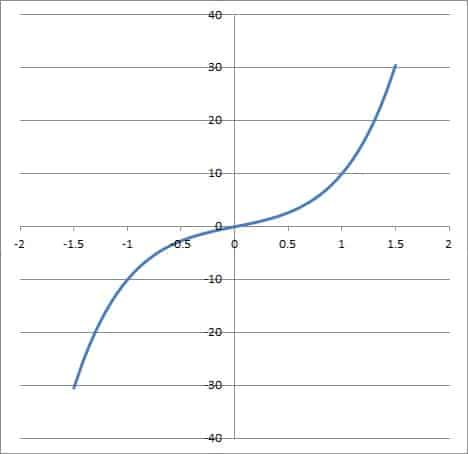The quintic function f(x) = x5 + 5x3 + 4x has zeros at x = 0, i, -i, 2i, and -2i. However, only the real zero at x = 0 shows up on the graph.

Note that the function approaches negative infinity as x becomes more negative.  The function approaches positive infinity as x becomes more positive.

## Can You Factor A Quintic Function?

You can factor a quintic function, but it can be challenging in some cases.  One potentially useful method is to factor by grouping.

Since a quintic has up to 6 terms, we would have to consider 3 pairs of terms to factor by grouping.  Let’s look at an example to see how it would work.

### Example 1: Factor A Quintic Polynomial By Grouping

Consider the quintic function

• f(x) = x5 + x4 – 13x3 – 13x2 + 36x + 36

There is no GCF to factor out.

Let’s break this into three groups of 2 terms each: the first 2, the middle 2, and the last 2:

• f(x) = (x5 + x4) + (- 13x3 – 13x2) + (36x + 36)

We can factor out x4 from the first set of parentheses:

• f(x) = x4(x + 1) + (- 13x3 – 13x2) + (36x + 36)

We can also factor out -13x2 from the second set of parentheses:

• f(x) = x4(x + 1) – 13x2(x + 1) + (36x + 36)

In addition, we can factor out 36from the third set of parentheses:

• f(x) = x4(x + 1) – 13x2(x + 1) + 36(x + 1)

Now we have the common term (x + 1) in all three expressions, so we factor it out:

• f(x) = (x + 1)(x4 – 13x2 + 36)

We can also factor the trinomial (in the second parentheses) as a product of two binomials:

• f(x) = (x + 1)(x2 – 4)(x2 – 9)

Now we can factor both the second and third parentheses as differences of squares:

• f(x) = (x + 1)(x + 2)(x – 2)(x + 3)(x – 3)

The quintic is now completely factored.  These 5 linear factors tells us that the zeros of the original quintic are x = 1, 2, -2, 3, and -3.

You can see the graph of this function below.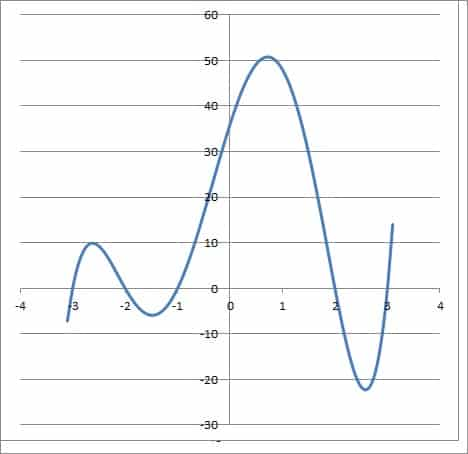The quintic function f(x) = x5 + x4 – 13x3 – 13x2 + 36x + 36 has zeros at x =1, 2, -2, 3, and -3.

### Example 2: Factor A Quintic Polynomial By Grouping

Consider the quintic function

• f(x) = 3x5 – 48x

First, we factor out the GCF of 3x:

• f(x) = 3x(x4 – 16)

Then, we factor the binomial in parentheses as a difference of squares:

• f(x) = 3x(x2 + 4)(x2 – 4)

Then, we factor the binomial in the second parentheses, again using a difference of squares:

• f(x) = 3x(x2 + 4)(x + 2)(x – 2)

This polynomial is now factored completely over the real numbers.  However, if we factor over the set of complex numbers, we get:

• f(x) = 3x(x+ 2i)(x – 2i)(x + 2)(x – 2)

The quintic is now completely factored.  These 5 linear factors tells us that the zeros of the original quintic are x = 0, 2, -2, 2i, and -2i.

You can see the graph of this function below.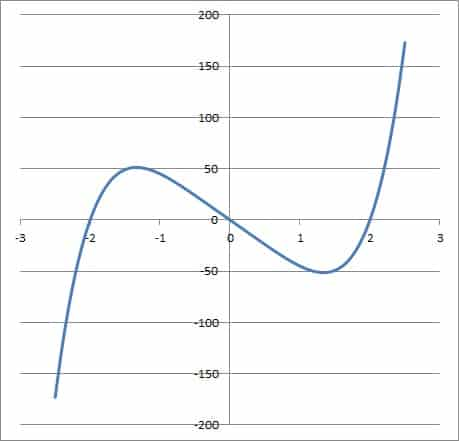The quintic function f(x) = x5 – 48x has zeros at x =0, 2, -2, 2i, and -2i. The imaginary roots 2i and -2i do not appear on the graph.

## How Many Zeros Can A Quintic Function Have?

A quintic function has 5 zeros – no more and no less.  Some of these zeros may be complex or imaginary numbers, while others may be real numbers.

According to the Fundamental Theorem of Algebra, a polynomial of degree n with real coefficients has n complex roots (counting repeated roots).  Applying this to a quintic with real coefficients (n = 5), we can see that such a function has 5 roots (possibly repeated roots).

A quintic function can have imaginary roots in some cases.  A quintic function will always have 0, 2, or 4 imaginary roots, which must be complex conjugates of one another (according to the Complex Conjugate Root Theorem).

For example, if x = 2i is a root of a quintic f(x), then x = -2i (the complex conjugate of 2i) is also a root of f(x).

This implies that a quintic function has either 5, 3, or 1 real roots. A polynomial of odd degree can never have an even number of real roots.

The table below summarizes the possibilities for real and complex or imaginary roots of quintic functions.

## Conclusion

Now you know more about quintic functions, their roots, and what their graphs look like.  You also know a little more about their symmetry.